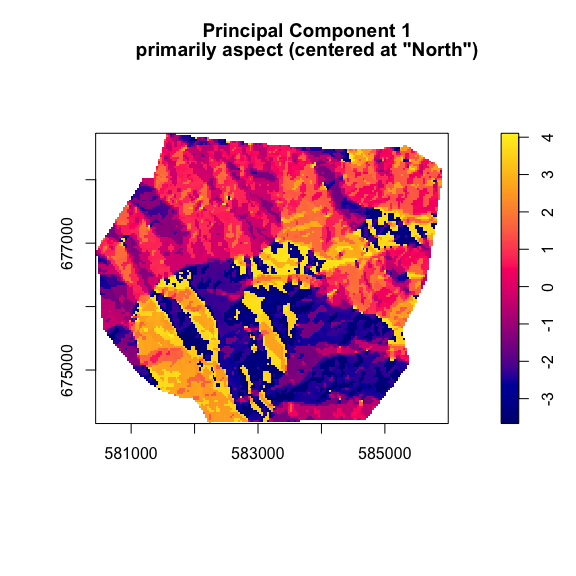# envi: Environmental Interpolation using Spatial Kernel Density Estimation

#### 2022-08-25

Start with the necessary packages and seed for the vignette.

``````loadedPackages <- c("envi", "maptools", "raster", "RStoolbox", "spatstat.data", "spatstat.geom", "spatstat.random")
invisible(lapply(loadedPackages, library, character.only = TRUE))
set.seed(1234) # for reproducibility``````

We use the `gorillas` data and the accompanying covariate data in `gorillas.extra` from the `spatstat.data` package on CRAN. These data are locations of nesting sites of gorillas in the Kagwene Gorilla Sanctuary in Cameroon. A detailed description and analysis of the data are reported in Funwi-Gabga and Mateu (2012). The authors used a kernel density-based smoothing technique to detect hot spots of nesting in the park. Here, we use another kernel density-based smoothing technique to detect hot spots of nesting within the covariate information (i.e., the gorilla ecological niche) and then predict where these hot spots are located within the park.

### Data preparation for lrren

We start by importing the two covariate data of class `im`:

1. Slope gradient (degrees)
2. Distance from water (meters)
``````slopeangle <- spatstat.data::gorillas.extra\$slopeangle
waterdist <- spatstat.data::gorillas.extra\$waterdist``````

Center and scale the covariate data.

``````slopeangle\$v <- scale(slopeangle)
waterdist\$v <- scale(waterdist)``````

Convert the covariate data to class `RasterLayer`.

``````slopeangle_raster <- raster::raster(slopeangle)
waterdist_raster <- raster::raster(waterdist)``````

Add appropriate marks to the `gorillas` data from `spatstat.data` package. These points are considered our “presence” locations.

``````presence <- spatstat.geom::unmark(spatstat.data::gorillas)
spatstat.geom::marks(presence) <- data.frame("presence" = rep(1, presence\$n),
"lon" = presence\$x,
"lat" = presence\$y)
spatstat.geom::marks(presence)\$slopeangle <- slopeangle[presence]
spatstat.geom::marks(presence)\$waterdist <- waterdist[presence]``````

Randomly draw points from the study area and add the appropriate marks. These points are considered our “(pseudo-)absence” locations.

``````absence <- spatstat.random::rpoispp(0.00004, win = slopeangle)
spatstat.geom::marks(absence) <- data.frame("presence" = rep(0, absence\$n),
"lon" = absence\$x,
"lat" = absence\$y)
spatstat.geom::marks(absence)\$slopeangle <- slopeangle[absence]
spatstat.geom::marks(absence)\$waterdist <- waterdist[absence]``````

Combine the presence (n = 647) and absence (769) locations into one object of class `data.frame` and reorder the features required for the `lrren` function in the `envi` package:

1. ID
2. X-coordinate
3. Y-coordinate
4. Presence (binary)
5. Covariate 1
6. Covariate 2
``````obs_locs <- spatstat.geom::superimpose(absence, presence, check = FALSE)
spatstat.geom::marks(obs_locs)\$presence <- as.factor(spatstat.geom::marks(obs_locs)\$presence)
spatstat.geom::plot.ppp(obs_locs,
which.marks = "presence",
main = "Gorilla nesting sites (red-colored)\nPseudo-absence locations (blue-colored)",
cols = c("#0000CD","#8B3A3A"),
pch = 1,
axes = TRUE,
ann = TRUE)
obs_locs <- spatstat.geom::marks(obs_locs)
obs_locs\$id <- seq(1, nrow(obs_locs), 1)
obs_locs <- obs_locs[ , c(6, 2, 3, 1, 4, 5)]``````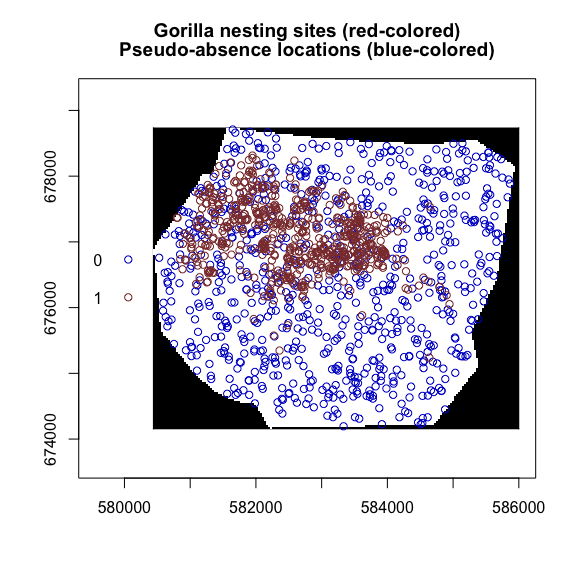Extract the prediction locations within the study area from one of the covariates.

``````predict_locs <- data.frame(raster::rasterToPoints(slopeangle_raster))
predict_locs\$layer2 <- raster::extract(waterdist_raster, predict_locs[, 1:2])``````

### lrren with two covariates

Run the `lrren` function within the `envi` package. We use the default settings, except we want to predict the ecological niche within the study area (`predict = TRUE`), so we conduct k-fold cross-validation model fit diagnostics (`cv = TRUE`) by undersampling absence locations to balance the prevalence (0.5) within all testing data sets (`balance = TRUE`).

``````start_time <- Sys.time() # record start time
test_lrren <- lrren(obs_locs = obs_locs,
predict_locs = predict_locs,
predict = TRUE,
cv = TRUE,
balance = TRUE)
end_time <- Sys.time() # record end time
lrren_time <- end_time - start_time # calculate duration of lrren() example``````

A single run of the `lrren` function took approximately 2.8 seconds on a machine with the features listed at the end of the vignette.

We display the estimated ecological niche within a space of Covariate 1 by Covariate 2 using the `plot_obs` function. We use the default two-tailed alpha level (`alpha = 0.05`) and the default colors where the yellow color denotes areas with covariate data combinations where we have sparse observations. As expected, extreme values of the log relative risk surface are located near the edges of the surface; however, these areas are highly variable and are not statistically significant based on an asymptotic normal assumption. The default color key for the log relative risk surface hides the heterogeneity closer to the null value (zero). Therefore, we limit the color key for the log relative risk surface to (-1, 1).

``````plot_obs(test_lrren,
lower_lrr = -1,
upper_lrr = 1)``````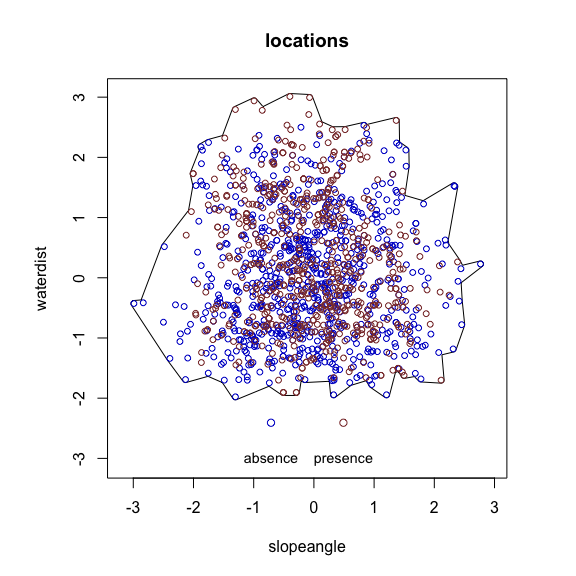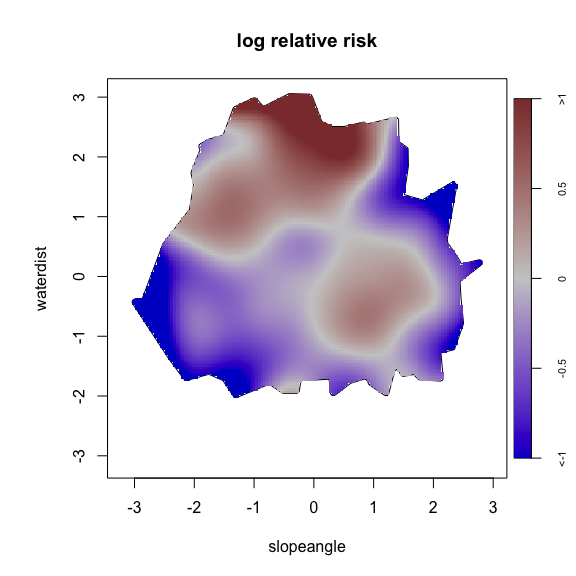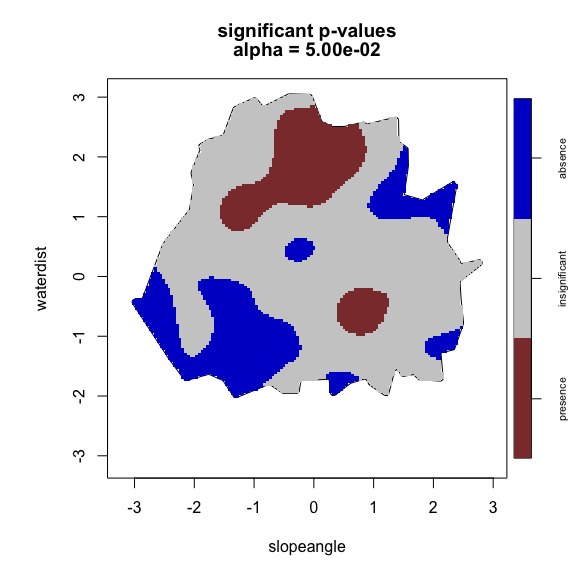We observe two areas of positive log relative risk, and the center of these areas are statistically significant, suggesting two ecological niches of gorilla nesting sites compared to pseudo-absence points drawn randomly from within the park (based on only two covariates, slope gradient and distance from water). One niche is a combination of flat to moderate (about 10 - 30 degrees) slope gradient and moderate to far (about 200 - 400 meters) distance from water, which may correspond to the top of ridges. The second niche is a combination of moderate (30 - 40 degrees) slope gradient and moderate (about 100 - 200 meters) distance from water, which may correspond to within valleys.

We display the estimated ecological niche predicted to the study area within geographic space using the `plot_predict` function. We use the default two-tailed alpha level (`alpha = 0.05`) and the default colors where the yellow color denotes areas with covariate data combinations where we have sparse observations. We limit the color key for the log relative risk prediction to (-1, 1).

``````plot_predict(test_lrren, cref0 = "EPSG:32632", cref1 = "EPSG:4326",
lower_lrr = -1,
upper_lrr = 1)``````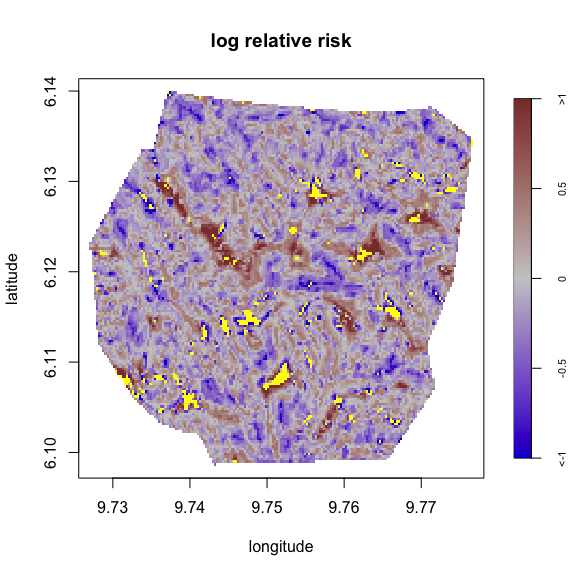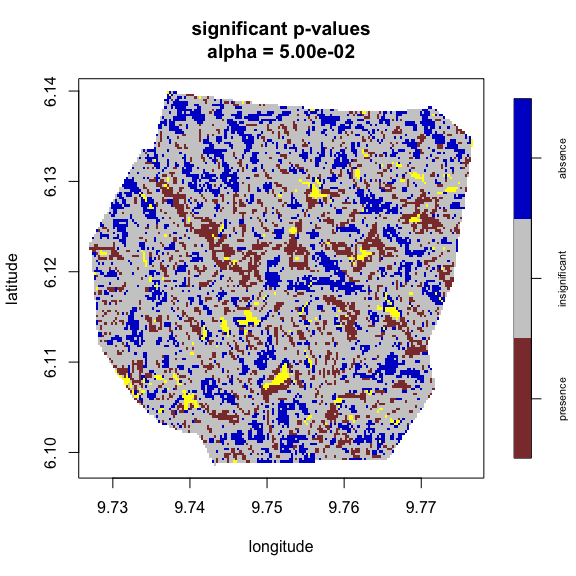The two estimated ecological niches are located in many small regions throughout the park, reflected by the large spatial heterogeneity in the two covariates. For example, the tops of ridges are the farthest distance from water and are located in many areas throughout the park. Importantly, gorilla nesting sites were not observed in many of these areas, but this prediction represents areas with combinations of covariates that match (or are similar) to the combinations occurring at observed locations.

This is an example of a scale mismatch. The scale of the gorilla nesting site presence is a broad, elliptical-shaped area in the northwest region of the park. Spatial interpolation of the gorilla nesting sites (i.e., not considering covariate information) can be seen in Figure 5a within the original study by Funwi-Gabga and Mateu (2012). The two covariates (slope gradient and distance from water) vary considerably within this area, and we observe a full range of covariate values within the larger gorilla presence area. Our approach is a version of environmental interpolation and considers covariate information when predicting the spatial distribution of gorilla nesting sites. When the gorilla nesting sites are arranged in covariate space, the smoothing bandwidth of our kernel density-based approach creates a smoother relative risk surface than the covariate surfaces themselves because the gorilla nesting sites are clustering in particular combinations of the two covariates (i.e., ecological niche) that are scattered throughout the park in geographic space.

We display the internal 10-fold cross-validation diagnostics using the `plot_cv` function. We use the default two-tailed alpha level (`alpha = 0.05`) and our prevalence is fairly balanced at 0.4569209.

``plot_cv(test_lrren)``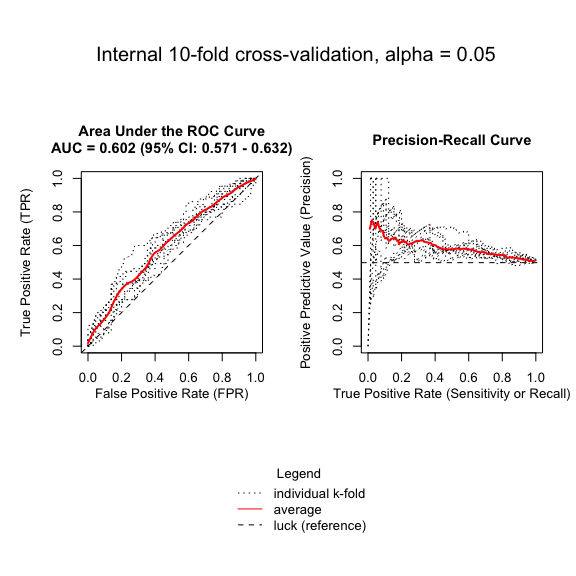The log relative risk estimate accurately predicts about 60% of the gorilla nesting sites, which is better than chance (50%) but not a large improvement. The pseudo-absence locations are drawn at random throughout the park and are located in areas estimated with higher log relative risk values, which reduces the prediction performance of the model. Using observed absences instead of pseudo-absences may improve the prediction of the spatial distribution of gorilla nesting sites.

### lrren with two principal components

The choice in covariates is critical for ecological niche models and especially for `lrren` because we are limited to two covariates. The goal, if possible, is to discover covariates that separate presence locations (i.e., nesting sites) from absence locations. Because our pseudo-absence locations are randomly drawn within the park it will be challenging to completely separate presence and absence locations in covariate space. One approach to include more than two covariates is to conduct an orthogonal linear transformation on multiple covariates, such as a Principal Component Analysis (PCA). Here, we can use the first two components of a PCA of all seven available covariates in the `gorillas.extra` data in the `spatstat.data` package, which include:

1. Aspect
2. Elevation (meters)
3. Heat index
5. Slope position
6. Vegetation type
7. Distance from water

We can use the `rasterPCA` function within the `RStoolbox` package to conduct a PCA of multiple spatial data layers. We start by centering and scaling the numeric-valued layers. We also center the factor-valued layer ‘aspect’ at “North” and we group flatter slope types together in the factor-valued layer ‘slope type.’ NOTE: Using categorical (discrete) variables in PCA is requires more consideration than demonstrated in this vignette (see: Kolenikov & Angeles (2009) for more details).

``````aspect <- spatstat.data::gorillas.extra\$aspect # class factor
elevation <- spatstat.data::gorillas.extra\$elevation # class integer
heat <- spatstat.data::gorillas.extra\$heat # class factor
slopeangle <- spatstat.data::gorillas.extra\$slopeangle # class numeric
slopetype <- spatstat.data::gorillas.extra\$slopetype # class factor
vegetation <- spatstat.data::gorillas.extra\$vegetation # class factor
waterdist <- spatstat.data::gorillas.extra\$waterdist # class numeric

# Center and scale numeric
elevation\$v <- scale(elevation\$v)
slopeangle\$v <- scale(slopeangle\$v)
waterdist\$v <- scale(waterdist\$v)

# Create rasters
aspect <- raster::raster(aspect)
elevation <- raster::raster(elevation)
heat <- raster::raster(heat)
slopeangle <- raster::raster(slopeangle)
slopetype <- raster::raster(slopetype)
vegetation <- raster::raster(vegetation)
waterdist <- raster::raster(waterdist)

# Reorder aspect to center by "N" instead of "S"
raster::values(aspect) <- factor(values(aspect),
levels = c("5", "6", "7", "8", "1", "2", "3", "4"))

# Reorder slope types to order flatter types next to each other
raster::values(slopetype) <- factor(values(slopetype),
levels = c("1", "6", "3", "2", "4", "5"))

# Save names for RasterLayers
names(aspect) <- "aspect"
names(elevation) <- "elevation"
names(heat) <- "heat"
names(slopeangle) <- "slopeangle"
names(slopetype) <- "slopetype"
names(vegetation) <- "vegetation"
names(waterdist) <- "waterdist"

# RasterStack
park <- raster::stack(aspect, elevation, heat, slopeangle, slopetype, vegetation, waterdist)

# Principal Component Analysis
pca_park <- RStoolbox::rasterPCA(park)
summary(pca_park\$model) # PCA components``````
``````## Importance of components:
##                           Comp.1    Comp.2    Comp.3     Comp.4     Comp.5
## Standard deviation     2.1697414 1.5626701 1.5429105 1.00679778 0.97729569
## Proportion of Variance 0.3786484 0.1964060 0.1914704 0.08152761 0.07681962
## Cumulative Proportion  0.3786484 0.5750545 0.7665249 0.84805249 0.92487211
##                            Comp.6     Comp.7
## Standard deviation     0.81117157 0.52542744
## Proportion of Variance 0.05292315 0.02220474
## Cumulative Proportion  0.97779526 1.00000000``````

The first two components of the PCA explain over 57% of the variation across the seven covariates.

``pca_park\$model\$loadings # PCA loadings``
``````##
##            Comp.1 Comp.2 Comp.3 Comp.4 Comp.5 Comp.6 Comp.7
## aspect      0.994
## elevation         -0.373 -0.179  0.276         0.864
## heat                                                  0.998
## slopeangle                       0.611  0.767 -0.147
## slopetype         -0.482  0.872
## vegetation        -0.773 -0.436 -0.294  0.118 -0.322
## waterdist         -0.155         0.678 -0.618 -0.357
##
##                Comp.1 Comp.2 Comp.3 Comp.4 Comp.5 Comp.6 Comp.7
## SS loadings     1.000  1.000  1.000  1.000  1.000  1.000  1.000
## Proportion Var  0.143  0.143  0.143  0.143  0.143  0.143  0.143
## Cumulative Var  0.143  0.286  0.429  0.571  0.714  0.857  1.000``````

The loadings of the first component is almost entirely the centered ‘aspect’ variable. The loadings of the second component is a combination of ‘vegetation type,’ centered ‘slope type,’ centered ‘elevation,’ and centered ‘distance from water.’

``````# Extract Bands from PCA
pca_bands <- pca_park\$map
pc1 <- pca_bands[] # PC1
pc2 <- pca_bands[] # PC2
pc1 <- spatstat.geom::as.im(pc1) # convert to class 'im'
pc2 <- spatstat.geom::as.im(pc2) # convert to class 'im'
spatstat.geom::plot.im(pc1,
main = 'Principal Component 1\nprimarily aspect (centered at "North")',
ann = TRUE,
axes = TRUE)
spatstat.geom::plot.im(pc2,
main = 'Principal Component 2\ncombination of vegetation type, slope type,\nelevation, and distance from water',
ann = TRUE,
axes = TRUE)``````